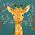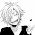## Rein's Proportion Problem

http://www.ikea.com/us/en/catalog/products/70152467/

You estimate that you can do 12 math problems in 45 min. How long should it take you to do 20 math problems?

Let x be the minute for 20 question.
Word ratio, Ratio tables, find x

Mins       Math problem                                      12(x) = 45(20)
45              12                                                    12 x/12 = 900/12
x               20                                                     X = 75 min.

It take 75 mins to do 20 math Problems.

http://blog.deezer.com/nl/deezer-music-poster-factory/

Three poster cost \$9.60. At the rate how many posters can you buy for \$48?

Let x be the number of poster that cost \$48
Word ratio, Ratio tables, find X

Poster       \$                                9.60(x) = 3(48)
3           9.60                             9.60 x/9.60= 144/9.60
X           48                                 X = 15 posters

You can buy 15 posters  for \$48.

which cost more per issue an 18 issue subscription for \$40.50 or a 12 issue subscription for \$33.60?

2:17 PM

#### 2 comments :

1.~Issue that cost more is the 12 subscription issue because:
12•x = 33.60•1
12x = 33. 60
12 12
x=2.80
While the other one is cheaper:
18•x = 40.50•1
18x = 40.50
18 18
x=2.25

2.Justine how did you answer it with dots?